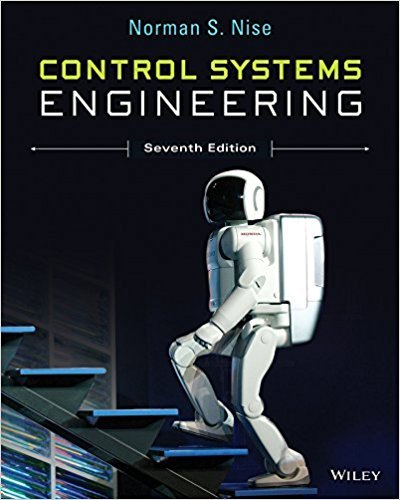×
Get Full Access to Control Systems Engineering - 7 Edition - Chapter 7 - Problem 64
Get Full Access to Control Systems Engineering - 7 Edition - Chapter 7 - Problem 64

×

# Packet information flow in a router working under TCP/IPISBN: 9781118170519 162

## Solution for problem 64 Chapter 7

Control Systems Engineering | 7th Edition

• Textbook Solutions
• 2901 Step-by-step solutions solved by professors and subject experts
• Get 24/7 help from StudySoup virtual teaching assistantsControl Systems Engineering | 7th Edition

4 5 1 244 Reviews
29
5
Problem 64

Packet information flow in a router working under TCP/IP can be modeled using the linearized transfer function P s Q s f s C2 2N esR s 2N R2C s 1 R where C = link capacity (packets/second) N = load factor (number of TCP sessions) Q = expected queue length R = round trip time (second) p =probability of a packet drop The objective of an active queue management (AQM) algorithm is to automatically choose a packet-drop probability, p, so that the queue length is maintained at a desired level. This system can be represented by the block diagram of Figure P7.13 with the plant model in the P(s) block, the AQM algorithm in the G(s) block, and F s H s 1. Several AQM algorithms are available, but one that has received special attention in the literature is the random early detection (RED) algorithm. This algorithm can be approximated with G s LK s K, where L and K are constants (Hollot, 2001). Find the value of L required to obtain a 10% steady-state error for a unit step input when C= 3750 packets/s, N=50 TCP sessions, R= 0.1 s, and K= 0.005.

Step-by-Step Solution:
Step 1 of 3

Software Copyrights: Early days: • Hardware manufacturers made their own software o Worked just on their machine since there weren’t really competing manufacturers • 70s - emergence of standalone/independent software companies • copyright act of 1976 o recognizes ability to copyright software § creative expression is protected § concept is licensing use not ownership of item Lotus v. Borland- US Supreme Court (1996) • Quattro Pro (Borland) o Replicated experience of Lotus’ 1-2-3 o Borland didn’t copy any lines of code from Lotus 1-2-3 o However did work to create a similar experience § Menus and shortcuts were same o Was this copyright infringe

Step 2 of 3

Step 3 of 3

##### ISBN: 9781118170519

The full step-by-step solution to problem: 64 from chapter: 7 was answered by , our top Engineering and Tech solution expert on 11/23/17, 05:05AM. The answer to “Packet information flow in a router working under TCP/IP can be modeled using the linearized transfer function P s Q s f s C2 2N esR s 2N R2C s 1 R where C = link capacity (packets/second) N = load factor (number of TCP sessions) Q = expected queue length R = round trip time (second) p =probability of a packet drop The objective of an active queue management (AQM) algorithm is to automatically choose a packet-drop probability, p, so that the queue length is maintained at a desired level. This system can be represented by the block diagram of Figure P7.13 with the plant model in the P(s) block, the AQM algorithm in the G(s) block, and F s H s 1. Several AQM algorithms are available, but one that has received special attention in the literature is the random early detection (RED) algorithm. This algorithm can be approximated with G s LK s K, where L and K are constants (Hollot, 2001). Find the value of L required to obtain a 10% steady-state error for a unit step input when C= 3750 packets/s, N=50 TCP sessions, R= 0.1 s, and K= 0.005.” is broken down into a number of easy to follow steps, and 195 words. This textbook survival guide was created for the textbook: Control Systems Engineering, edition: 7. Since the solution to 64 from 7 chapter was answered, more than 447 students have viewed the full step-by-step answer. This full solution covers the following key subjects: Algorithm, Block, TCP, Packet, aqm. This expansive textbook survival guide covers 13 chapters, and 734 solutions. Control Systems Engineering was written by and is associated to the ISBN: 9781118170519.

Unlock Textbook Solution